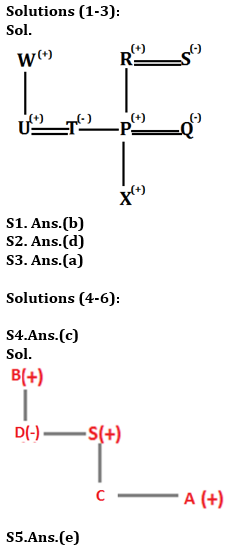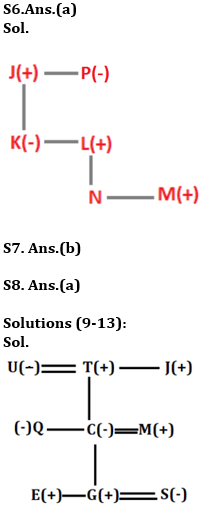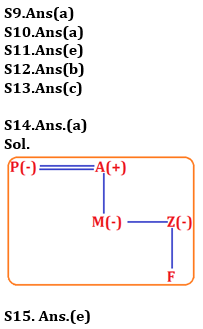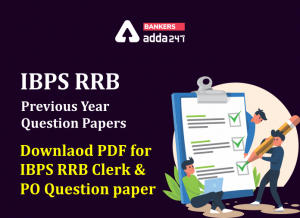Latest Banking jobs   »   Reasoning Ability, Blood Relation Quiz For...

# Reasoning Ability, Blood Relation Quiz For IBPS RRB PO, Clerk Prelims 2021- 17th June

Directions (1-3): Study the following information carefully to answer the given questions.
Eight Persons P, Q, R, S, T, U, W and X in a family. There are three married couple. T is sister P. R is grandfather of X. W is father of U. Q is daughter in law of S who is not married to W. X is unmarried male and T is married to U who has no child.

Q1. Who among the following is nephew of T?
(a) R
(b) X
(c) S
(d) Q
(e) None of these

Q2. Who among the following is son in law of S?
(a) P
(b) X
(c) R
(d) U
(e) None of these

Q3. What is the relation of Q with X?
(a) Mother
(b) Aunt
(c) Grand-Mother
(d) Sister
(e) Wife

Directions (4-6): Study the following information and answer the questions given below:
‘Q + R’ means ‘Q is father of R’
‘Q ÷ R’ means ‘R is brother of Q’
‘Q × R’ means ‘Q is husband of R’
‘Q – R’ means ‘Q is sister of R’

Q4. In the expression B + D – S + C ÷ A, how is A related to B?
(a) Son
(b) Daughter
(c) Grandson
(d) Can’t be determined
(e) None of these

Q5. Which of the following expressions shows that Z is sister of X?
(a) J + Z– L + N ÷ X
(b) J – N – L ÷ X × Z
(c) J x L – Z÷ X + N
(d) J – Z– L + N ÷ X
(e) None of these

Q6. Which of the following is true for the given expression?
‘P- J + K – L + N ÷ M’
(a) P is aunt of N’s father
(b) K is father of M
(c) N is sister of M
(d) J is father of N
(e) None of these

Q7. Pointing to a photograph of a girl Rohit says, “She is the only daughter of my father.” How is Rohit related to the girl?
(a) Son
(b) Brother
(c) Father
(d) Uncle
(e) Nephew

Q8. Shivani says pointing towards a Girl, “She is the daughter of the wife of my brother”. In this relation, Shivani is a female then how Shivani is related to the girl?
(a) Aunt
(b) Father
(c) Uncle
(d) Grandfather
(e) Sister

Direction (9-13): Study the following information carefully and answer the given questions.
There are nine members in three generation family i.e. J, T, U, C, M, Q, E, S, and G. Among them there are 3 married couples and only four females. J is paternal uncle of Q. U has only 2 children. S is daughter-in-law of M and M is son-in-law of T. C is married to M. E and G are sons of C. E is not married. T is father in law of S’s father-in-law. T is married to U.

Q9. Who among the following person is father of Q?
(a) T
(b) U
(c) G
(d) S
(e) M

Q10. How is G related to T?
(a) Grandson
(b) Daughter
(c) Daughter in law
(d) Sister
(e) None of these

Q11. Which of the following is true about U?
(a) U is aunt of Q
(b) M is daughter of U
(c) J is sister of U
(d) S is grandson of U
(e) None of true

Q12. How S is related to son in law of T?
(a) Father
(b) Daughter in law
(c) Son
(d) Sister in law
(e) Wife

Q13. How M’s sister in law is related to J?
(a) Son in law
(b) Nephew
(c) Niece
(d) Daughter in law
(e) Sister in law

Directions (14-15): Study the following information carefully and answer the questions which follow:
‘M + K’ means ‘M is brother of K’
‘M ÷ K’ means ‘M is father of K’
‘M × K’ means ‘M is wife of K’
‘M – K’ means ‘M is sister of K’
‘M = K’ means ‘M is mother of K’

Q14. In expression ‘P × A ÷ M – Z = F’, how is F related to P?
(a) Grandchild
(b) Grandson
(c) Grand daughter
(d) Grandmother
(e) Son

Q15.Which of the following expression represents the relationship ‘D is daughter – in – law of C’?
(a) D × H + N = E ÷ C
(b) H + D × N ÷ E = C
(c) C = F + L – Z × D
(d) C × F ÷ L ÷ Z × D
(e) None of these

Practice More Questions of Reasoning for Competitive Exams:

###### Study Plan for IBPS RRB PO/Clerk Prelims 2021

Solutions#### Congratulations!Incorrect details? Fill the form again here

•Reasoning Ability Quiz For IBPS RRB PO C...
•Quantitative Aptitude Quiz For IBPS RRB ...
•Reasoning Ability Quiz For IBPS RRB PO C...
•Quantitative Aptitude Quiz For IBPS RRB ...
•IBPS RRB Previous Year Question Paper PD...
•Quantitative Aptitude Quiz For IBPS RRB ...